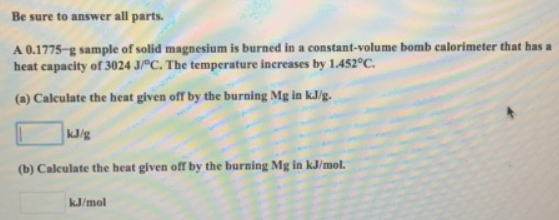# Problem: Be sure to answer all parts.A 0.1775-g sample of solid magnesium is burned in a constant-volume bomb calorimeter that has a heat capacity of 3024 J/°C. The temperature Increases by 1.452°C. (a) Calculate the heat given off by the burning Mg in kJ/g. (b) Calculate the heat given off by the burning Mg in kJ/mol.

###### FREE Expert Solution
90% (144 ratings)###### Problem Details

Be sure to answer all parts.

A 0.1775-g sample of solid magnesium is burned in a constant-volume bomb calorimeter that has a heat capacity of 3024 J/°C. The temperature Increases by 1.452°C.

(a) Calculate the heat given off by the burning Mg in kJ/g.

(b) Calculate the heat given off by the burning Mg in kJ/mol.